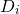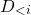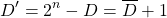# [CSCI150] Lecture 3: Combinational Circuits Design

Lecture 2 deals mainly with Boolean Algebra, the theoretical arsenal for circuit design. In Lecture 3, we will learn more practical design procedures, as well as functional blocks.

## LS9 Part 1 (Lecture): Systematic Designing Procedures, Curtain Controller Example

Highlight:

• 5 Step Systematic Designing Procedures
• Specification: (Provided, includes I/O ports and description in human language of the system)
• Formulation: transform spec into Truth Table or simple Boolean Expressions
• Optimisation: transform what you have in formulation into simplest forms (usually in boolean expressions, or later, functional blocks), so that it requires less gates and components in general to implement
• Technology Mapping:
• Technology: physical components you can use to implement, so far that includes gates and I/O devices (in LogicWorks, Binary Switch and Prob)
• Mapping: use available technology to implement your circuit
• Verification: test to see if your circuit meets the specification, or else go to debugging

## LS9 Part 2: Curtain Motor Controller Example in LogicWorks

Highlights:

• Steps
1. Do the I/O ports using binary switches and probs
2. Do the names of I/O ports according to your optimised boolean expressions
3. Start from the easiest connections, utilise the available technology and proceed gradually to the output
4. Test connections by clicking the wires always
5. Test the overall circuit (perform Verification essentially)

## LS10 Part 1: BCD-to-7-Segment-Display Example (Lecture + Tutorial)

Highlight

• LogicWorks components
• Hex Keyboard (use the one without STB port)
• 7 Segment Display
• AND/OR gates with more than 2 inputs (and some of them inverted)

## LS10 Part 2: Custom Components out of Existing Design (Tutorial)

Highlight:

• How to create a custom part
1. New Device Symbol
2. Options -> Sub-circuit and Part Type
3. Create sub-circuit symbol and select an open circuit to attach to it
4. Draw the box, and pins
5. Give it a name
7. Save the component in your library
8. Reward yourself some ice cream🍦

## LS10 Part 3: Hierarchical Design, 4-bit Equity Comparator (Lecture + Tutorial)

Highlight

• Hierarchical Design
• Divide and Conquer: break up a difficult problem into easier smaller problems, and solve
• Functional Blocks: a complete circuit in its own right, that you can easily reuse just like gates in other designs.
• In hierarchical designs, you can implement blocks functional blocks that are easier to do to save time
• Each block should also be defined using 5-step designing procedures
• Functional blocks are considered technology in Step4 of Systematic Designing Procedures

## LS10 Part 4: Few words on Tech Mapping & Auto Verification (Lecture)

Highlight:

• Technology Mapping
• Replacing everything with NAND and Inverters
• FPGA: Field Programmable Gating Array
• Programmable circuit boards
• You write the circuit in Hardware Description Language, then upload to the board. The board will then work like your designed circuit
• Not a von Neumann Computer
• Verification
• Manual & Auto: the latter uses computer assisted stuff

## LS11 Part 1: Elementary Functional Blocks (Lecture)

Highlights:

• Elementary Functional Blocks
1. Value Fixing: provide constant values of 0 and 1 to a wire
2. Value Transferring: provide the value of one wire to another
3. Inverting: NOT gate operation
4. Vector denotation: bus formulation (also called breakouts in LogicWorks)
5. Enabler: if EN signal is 1, works just like transferring for input X; if EN is 0, always output 0.

## LS11 Part 2: Elementary Functional Blocks (Tutorial)

Highlights:

• Implementing Elementary Functional Blocks
1. Value Fixing: use +5V for constant 1, digital ground for 0
2. Value Transferring: connect the wire, that’s it
3. Inverting: use the NOT gate
4. Vector denotation: use breakouts(busses) in LogicWorks, the thicker wire is used for connecting these busses
5. Enabler: an AND gate with input EN and X, outputs F

## LS11 Part 3: 1-to-2 and 2-to-4 Decoders (Lecture + Tutorial)

Highlights:

• 1-to-2 Decoder, and its implementation in logicworks
• 2-to-4 Decoder, and its implementation in logicworks
1. Using standard gates etc.
2. Using hierarchical design, with 1-to-2 decoder
3. Using 1-to-2 decoder and busses
• It is very important that you try to implement these on your own as well. Remember to create them in your library.

## LS11 Part 4: 3-to-8 and 4-to-16 Decoders (Lecture + Tutorial)

Highlights:

• 3-to-8 Decoder
• Using 2-to-4 Decoder, and busses
• 4-to-16 Decoder:
• Incremental design: use 3-to-8 decoder, and busses
• Recursive design: use (4/2 = 2)-to-4 decoder(s), and busses

## LS11 Part 5: 1-bit Binary Adder using Decoder (Lecture + Tutorial)

Highlights:

• Input (all 1 bit): X (addend), Y (augend), Z (previous carry)
• Output (all 1bit): S (sum), C (next carry)
• Use Sum-of-Minterm and decoder to implement with ease, and save as a component in your LogicWorks Library

## LS12: Enabler & Priority Enabler; Multiplexers

Highlights:

• Enabler: reverse function of decoders
• Problem: Invalid input leads to problems, including all 0 inputs and multiple 1s in the input
• Priority Enabler:
• Additional validity bit, equals 1 if input contains 1
• Priority:has higher priority than• Multiplexer
• Switching between multiple channels
• Each channel can have N-bits
• Switch signal (S) controls which input channel to output
• M-Channel N-bit Multiplexer can be designed using N x M-Channel 1-bit Multiplexers

## LS13: Full Adders (Lecture + Tutorial)

Highlights:

• Hierarchical design
• Simpler than using decoder

## LS14: Subtractors: Unsigned Binary Subtractor I (Lecture + Tutorial)

Highlights:

• Standard Unsigned Subtractor: X – Y, X >= Y
• 1-bit Subtractor using decoder
• n-bit Subtractor using 1-bit Subtractors

## LS15 Part 1: Unsigned 2s Complement, Adder-Subtractor (Lecture + Tutorial)

Highlights:

• Unsigned 2s Complementer
• Given n-bit binary integer (unsigned) D
• the unsigned 2s complement of D:• the unsigned 2s complement of D is equal to, bitwise invert of D plus 1
• Correcting X-Y when Y > X
• If the borrow bit output of your subtractor is 1, excessive values are not correctly subtracted from X, you need correction
• Correct output: negative unsigned 2s complement of output D
• Design a selective 2s complementer using Adder and XOR, plug into the subtractor
• Input vectors X, Y, Mode selector Subtract/notAdd
• Output vector D
• Mode Selector is 0, output X+Y
• Mode Selector is 1, output X-Y
• Use 2channel 4bit Multiplexer (4bit 2-to-1 Multiplexer) to implement

## LS15 Part 2: Subtraction using Adder

Highlights:

• Subtraction using unsigned 2s complement
• 2s comp of Y = 2^n – Y
• X – Y = X + (2^n – Y) – 2^n = X + Y’ – 2^n
• 2^n can be ignored because it is beyond n-bit
• XOR and Sub/notAdd input toggles between Y and Y’
• Output X + Y when Sub/notAdd = 0
• Output X + notY + 1 = X – Y when Sub/notAdd = 1

## LS16: Overflow, Signed Arithmetics, Other Functions

Highlights:

• Overflow, definition and how to use carry/borrow bit to detect
• Signed 2s Complement
• For signed integers only
• For positive, no change
• For negative, do NOT change the sign bit, all magnitude bits inverted and plus 1
• Signed Arithmetics (addition and subtraction Only)
• Step 1: Convert both signed numbers to signed 2s complement
• Step 2: Perform addition and subtraction using unsigned components
• Step 3: Convert result back using signed 2s complement
• Others
• Increment/Decrement
• Multiplier/Divisor by constant
• Others: extensions etc.

This site uses Akismet to reduce spam. Learn how your comment data is processed.